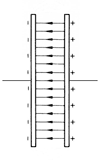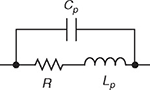What is resistor capacitance? Capacitance is an ability of a body to store electrical energy in the form of electrical charge. Practical resistors always exhibit capacitance as a parasitic property. Depending on the application, resistor capacitance might be easily disregarded, especially in DC circuits. In some applications, such as snubber resistors, the capacitive parasitic effect is actually a desirable effect. On the other hand, parasitic resistor capacitance can be a significant factor in high-frequency AC applications, creating an unwanted effect. The reason for this is that the impedance of a resistor rises with the applied voltage frequency due to the increase in its reactance. The higher the frequency, the lower the impedance is, which means that the resistor can no longer be observed as a constant element at high frequencies, and becomes a frequency-dependent element. Capacitors and resistors Electrical loads can be divided into two types: real (or resistive) loads and reactive loads. Real loads are used to convert electrical power into heat. An ideal resistor is a purely resistive load, which means that all the electrical power applied to the resistor is dissipated as heat. On the other hand, reactive loads convert electrical power into a magnetic or electric field and temporarily store it before returning it to the rest of the circuit. Reactive loads can be inductive or capacitive. Inductive load store energy in the form of a magnetic field, while capacitive loads store energy in the form of an electric field. The main difference between ideal resistors and ideal capacitors is therefore that resistors dissipate electrical power as heat, while capacitors turn electrical power into an electric field. Ideal resistors have zero reactance and as a result their capacitance is zero as well. Unfortunately, electrical devices are not ideal in practice and even the simplest resistors have [… read more]